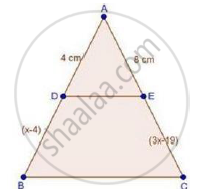Share

# In δAbc, D and E Are Points on the Sides Ab and Ac Respectively Such that De || Bc If Ad = 4, Ae = 8, Db = X – 4, and Ec = 3x – 19, Find X. - CBSE Class 10 - Mathematics

ConceptBasic Proportionality Theorem Or Thales Theorem

#### Question

In ΔABC, D and E are points on the sides AB and AC respectively such that DE || BC

If AD = 4, AE = 8, DB = x – 4, and EC = 3x – 19, find x.

#### SolutionWe have,

DE || BC

Therefore, by basic proportionality theorem, we have,

"AD"/"DB"="AE"/"EC"

4/(x-4)=8/(3x-19)

⇒ 4(3x – 19) = 8(x – 4)

⇒ 12x – 76 = 8x – 32

⇒ 12x – 8x = −32 + 76

⇒ 4x = 44

⇒ 𝑥 = 44/4 = 11 cm

∴ x = 11 cm

Is there an error in this question or solution?

#### Video TutorialsVIEW ALL 

Solution In δAbc, D and E Are Points on the Sides Ab and Ac Respectively Such that De || Bc If Ad = 4, Ae = 8, Db = X – 4, and Ec = 3x – 19, Find X. Concept: Basic Proportionality Theorem Or Thales Theorem.
S